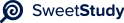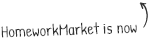•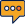0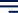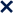1 / 7100%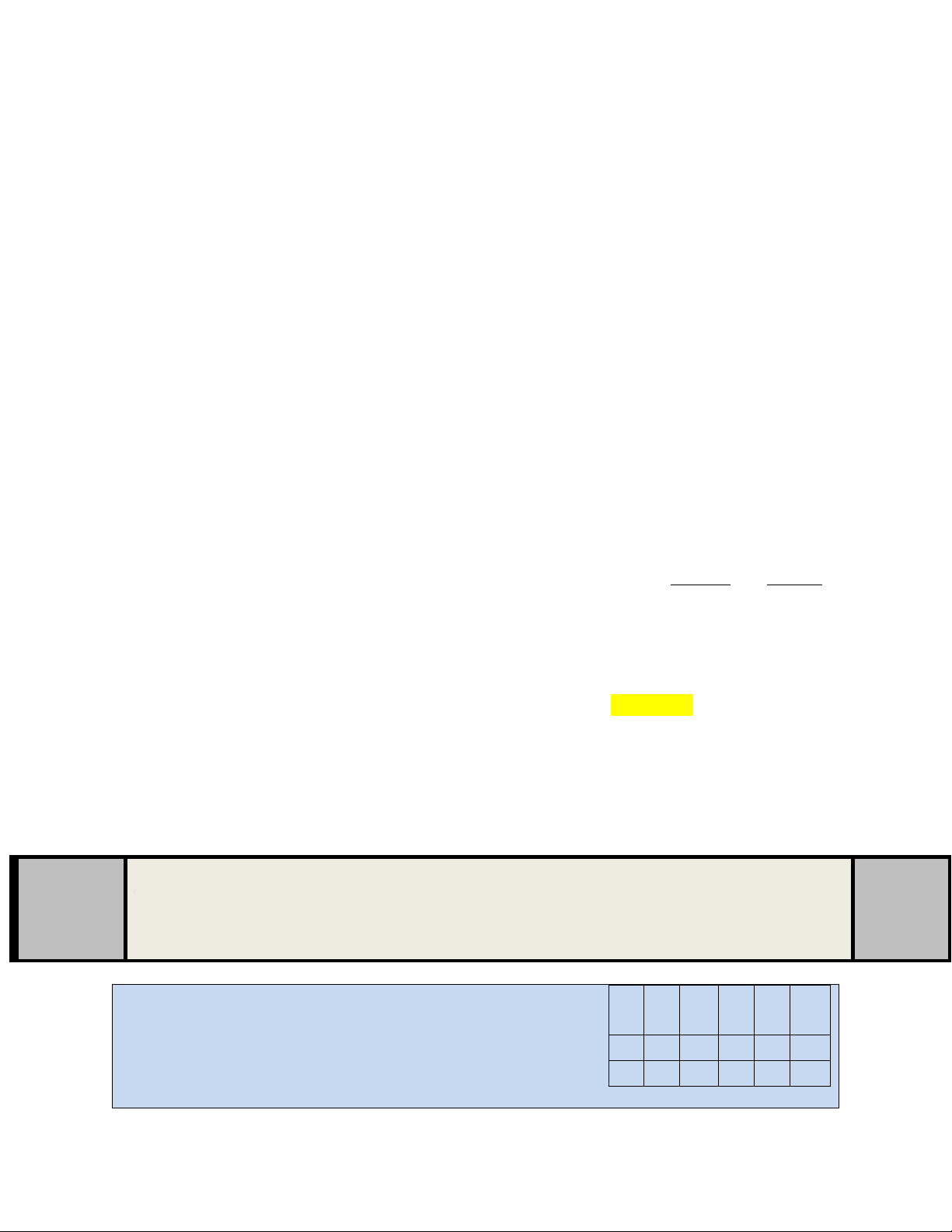PSYC 510
HOMEWORK: DATA ORGANIZATION AND DESCRIPTIVE STATISTICS
ASSIGNMENT INSTRUCTIONS
OVERVIEW
This Homework: Data Organization & Descriptive Statistics Assignment is designed to
assess your understanding of the concepts and applications covered thus far in this course. In this
module, you have looked at how to organize data and describe it in terms of central tendency,
dispersion, and shape of distribution. You also have covered cover how to standardize a
distribution of data in order to see how a single score compares to other scores. These topics are
covered conceptually as well as how to calculate them by hand and in SPSS. These concepts and
applications are fundamental to understanding and evaluating data as a consumer in a data-laden
world, a consumer of data within our field, and producer of research in our field.
INSTRUCTIONS
Be sure you have reviewed this moduleâs Learn section before completing this Homework:
Data Organization & Descriptive Statistics Assignment. This Homework: Data
Organization & Descriptive Statistics Assignment is worth 60 points. Each question is worth 3
points each. Six points are awarded for mechanics/structure.
ï· Part I contains general concepts from this moduleâs Learn section.
ï· Part II requires use of SPSS. You will have to take screen shots and/or copy and paste from
your SPSS to place answers within this file. Make sure you only insert relevant and legible
images.
ï· Part III is the cumulative section. These may include short answer and/or use of SPSS but
will review material from previous module(s).
ï· Directions for each subsection are provided in the top of each table (in the blue shaded
areas).
ï· Answers should be placed where indicated (wherever there is âANSWERâ).
ï· Submit the file as a WORD document (.doc or .docx). Make sure the filename of your
submission includes your full name, course and section.
o Example: HW4_JohnDoe_510B01
Make sure to check the Homework Grading Rubric before starting this Homework: Data
Organization & Descriptive Statistics Assignment.
Part I: General Concepts
These questions are based on the concepts covered in this
moduleâs assigned readings and presentations.
3
1
47 53 57 61 67
40
49 55 58 62 68
41
50 56 58 64 69
1. The following data represents 18 emotional intelligence test scores. Organize these
Page 1 of 7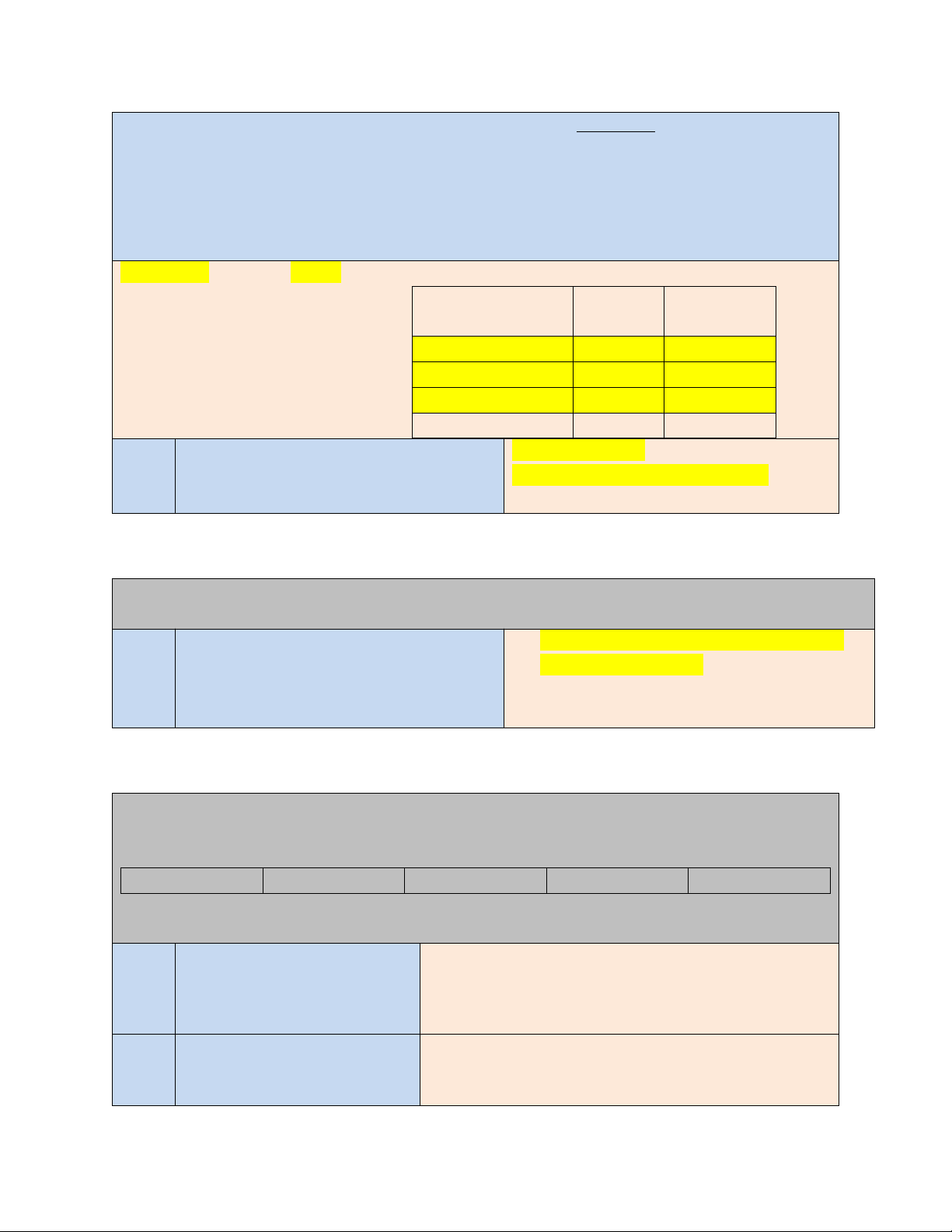PSYC 510
data into a class interval frequency distribution using 3 intervals with frequency (f) and
relative frequency (rf) columns.
ANSWER: Fill in the yellow cells:
2. What is the class width?
What is the upper class limit of the first
interval?
Class width is 13
Upper class limit of first clas is 44
Answer the following question related to your assigned readings and presentations.
3. What do the following symbols
represent?
a. N
b. Âµ
a. Number of Scores in a distribution
b. Population mean
Use the following five (5) numbers to calculate the following by hand (must show all work
and do NOT round)
3 3 4 5 9
4. Calculate all measures of
central tendency. Label each
one.
ANSWER Mean= (3+3+4+5+9)/ 5 =
4.8
Median= 4(Mid number)
Mode= 3
5. Is this data best described as
normal, positively skewed, or
negatively skewed? How does
It cannot be a normal distributuion
because the mean, median and mode are
not
Page 2 of 7
Class Intervals (use
whole numbers)
f rf (round to
2 decimals)
31-44 3 0.17
45-58 9 0.50
59-72 6 0.33
N = 18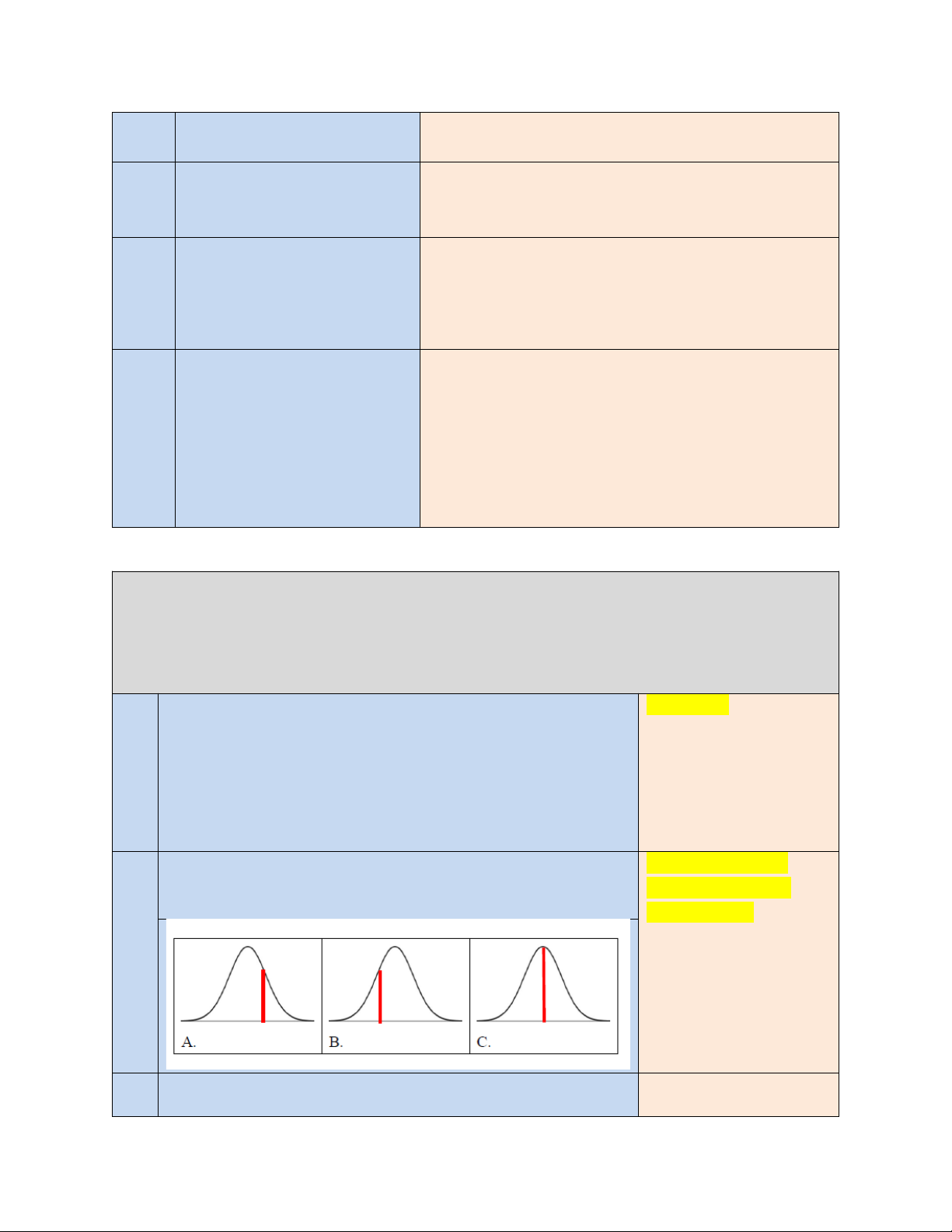PSYC 510
this?
equal.
6. Calculate the range and
average deviation. Show all
work.
Range= (9-3)=6
Average deviation= |(3-4.8) +(3-4.8)+ (4-4.8)+(5-4.8)
(9-4.8)|= 8.8 /5=average deviation of 1.76
7. Calculate the standard
deviation using the formula
from ch.5 . Show all work.
ANSWER (3-4.8)2 +(3-4.8)2+ (4-4.8)2+(5-4.8)2 +(9-
4.8)2= 21.884
Square root of 21.884 is 4.678
4.678/ 5-1= 1.1695
1.1695
8. State two ways the standard
deviation differs from the
average deviation.
1)Instead of using absolute vales of the
deviations to get rid of the negative, in
standard
deviations they are squared.
2)Standard deviation is
used in many other statistical procedures
where
average deviation is not.
Students in the psychology department consume an average of 5 cups of coffee per day with a standard
deviation of 1.75 cups. The number of cups of coffee consumed is normally distributed. Make sure
you do NOT round when answering any of these questions. When applicable, show ALL work
for full points.
9. Nancy drank 6 cups of coffee. What proportion of students
drank FEWER cups of coffee than Nancy? (do not round and
use the table in the appendix of the Jackson e-book)
Z =
(6-5)/1.75=0.571
4
Proportion of students
that drank fewer
=0.71567
10. Which of the following curves (A, B, or C) best illustrate
where Nancyâ s data would be?
Curve A as nancy
mean is 5 and she
drinks 6 cups
11. How many cups of coffee would an individual at the 80
th
Pr(Z<0.842)=0.8
5+0.842Ã1.75
Page 3 of 7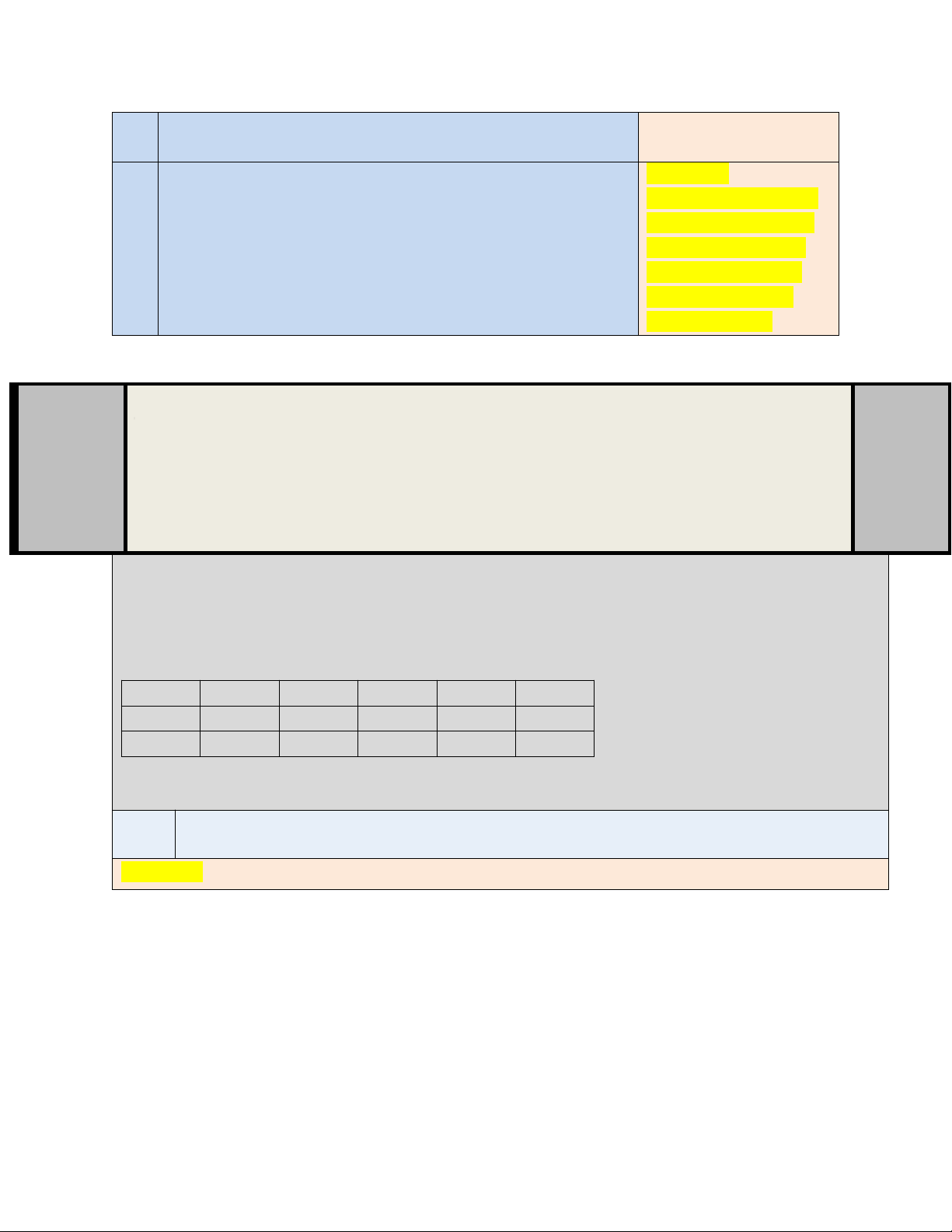PSYC 510
percentile rank drink? (do NOT round) =6.473 cups of coffee
12. Imagine this course is over and instead of seeing your total
points earned, you are given a z score and the grade is curved
to fit a normal distribution. What would you want your z
score to be and why? (make sure you use a numerical value
and indicate whether it is positive or negative)
I would want a Z score
of 3 and above as they
are extreme right end
and would give me a
larger area and thus
higher percentile
Part II: SPSS Application
These questions require the use of SPSS. Remember you must
submit all of your work within this word document. You will need
to take a screen shot of your data view if necessary, or copy and
paste your output into the spaces below.
The following dataset contains scores for depression, a subscale on the DASS-21 from 18
individuals. This variable is considered scale and is discrete. Enter the following data into SPSS to
answer the following questions. Make sure you name the variable âDepressionâ and that you set the
scale of measurement within SPSS.
11 13 15 17 18 22
7 8 18 17 13 11
9 16 18 12 13 15
13. Insert the descriptive statistics table (NOT the frequency table) from your SPSS output.
Page 4 of 7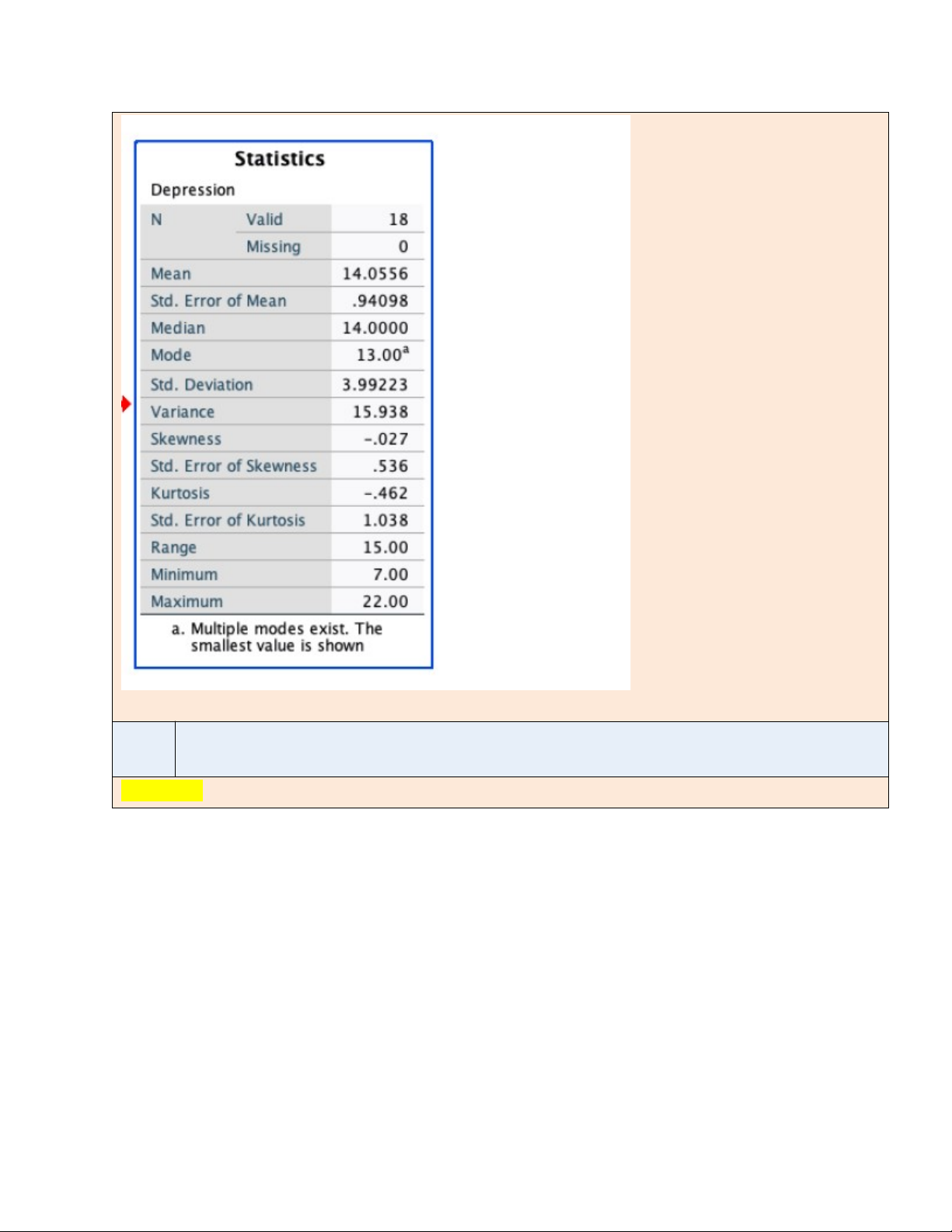PSYC 510
14. Create a histogram of the data using SPSS and paste it below.
Page 5 of 7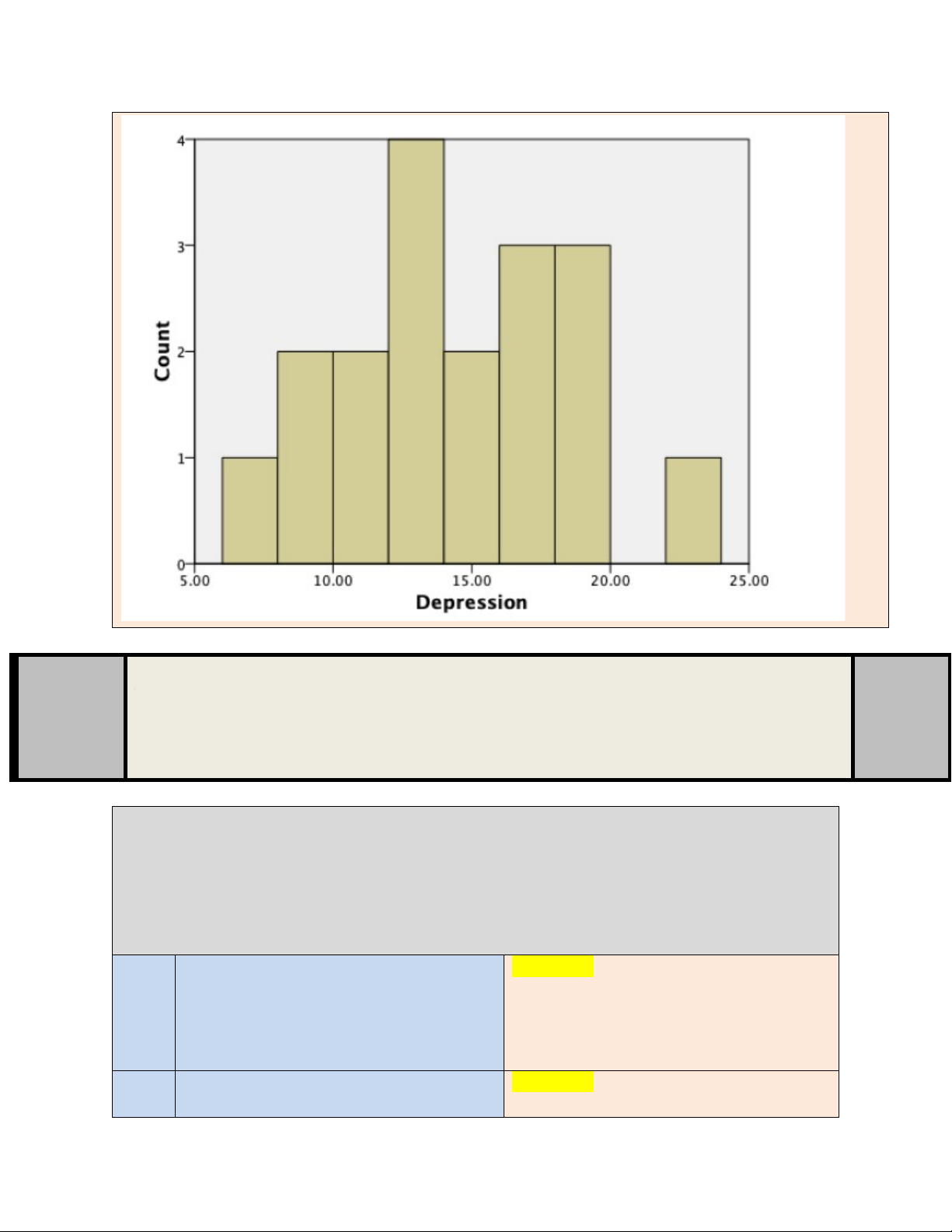PSYC 510
Part III: Cumulative
These questions can be related to anything covered thus far in the
course.
As part of his dissertation, Joe wanted to know if an icecream reward can increase the number
of books read by children in the first grade. The small school has four 1
st
grade classes â two
are given the reward and two are not; total number of books read at the end of the term are
compared.
15. What is the independent variable? Is it a
true manipulated variable or a subject /
participant variable?
The independent variable is
the reward given. It is a
manipulated variable .
16. What is the dependent variable? What is
its scale of measurement?
Dependent variable is the
Page 6 of 7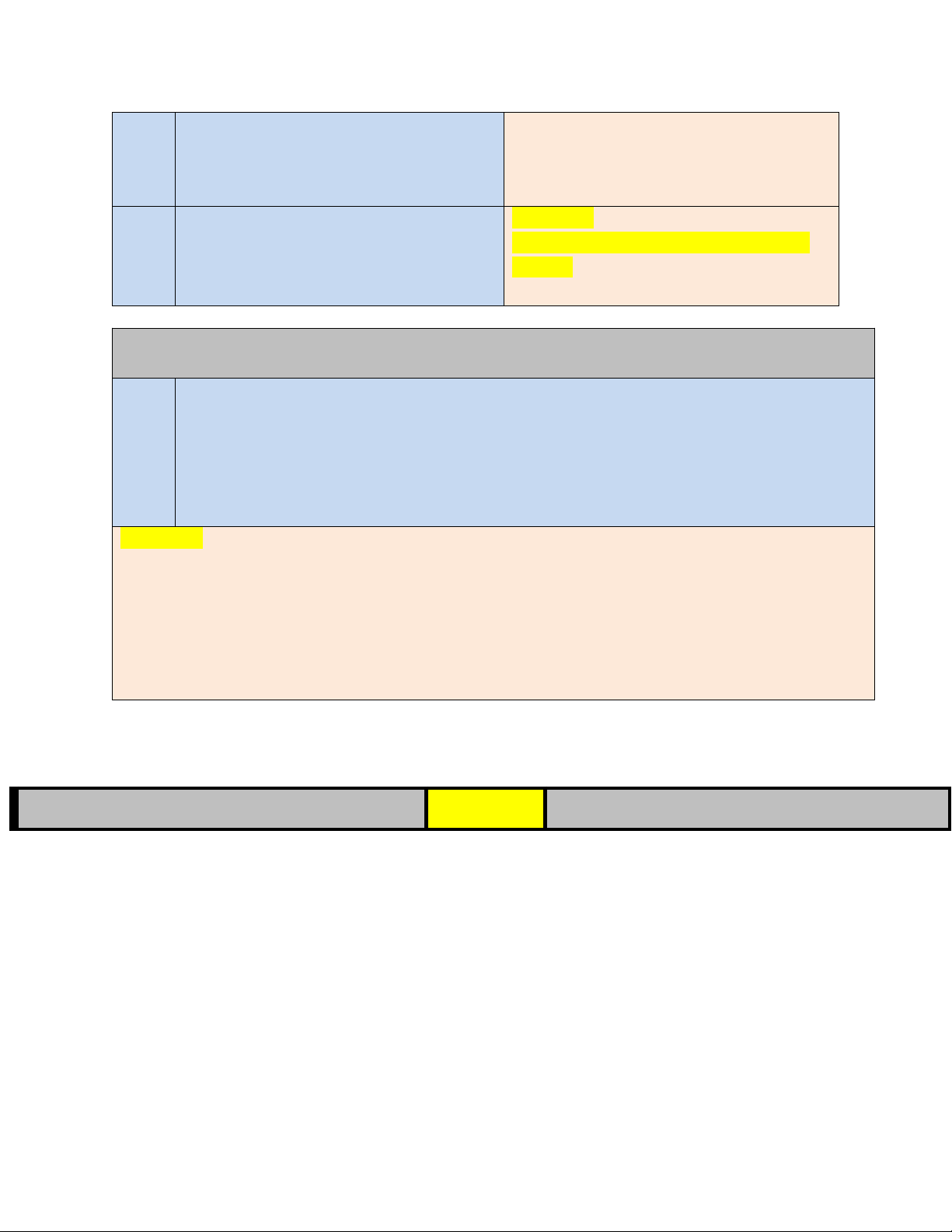PSYC 510
number of books read at the end
of
the term. Scale of measurement
is Ordinal
17. He found a huge difference â students
read more in the classes rewarded with
icecream. What is a potential
confounding variable?
Confounding variable can be number of
students
Answer the final question related to your previous readings and presentations on reliability and
validity.
18. When you log in to take your psychology exam, you are flabbergasted to find that all of the
questions are on calculus and not psychology. The next day in class, students complain so
much that the professor agrees to give you all a makeup exam the following day. When
you arrive in class the next day, you find that although the questions are different, they are
once again on calculus. In this example, there should be high reliability of what type?
What type(s) of validity is the test lacking? Explain your answers.